Ex 8.1

Chapter 8 Class 11 Binomial Theorem (Deleted)
Serial order wise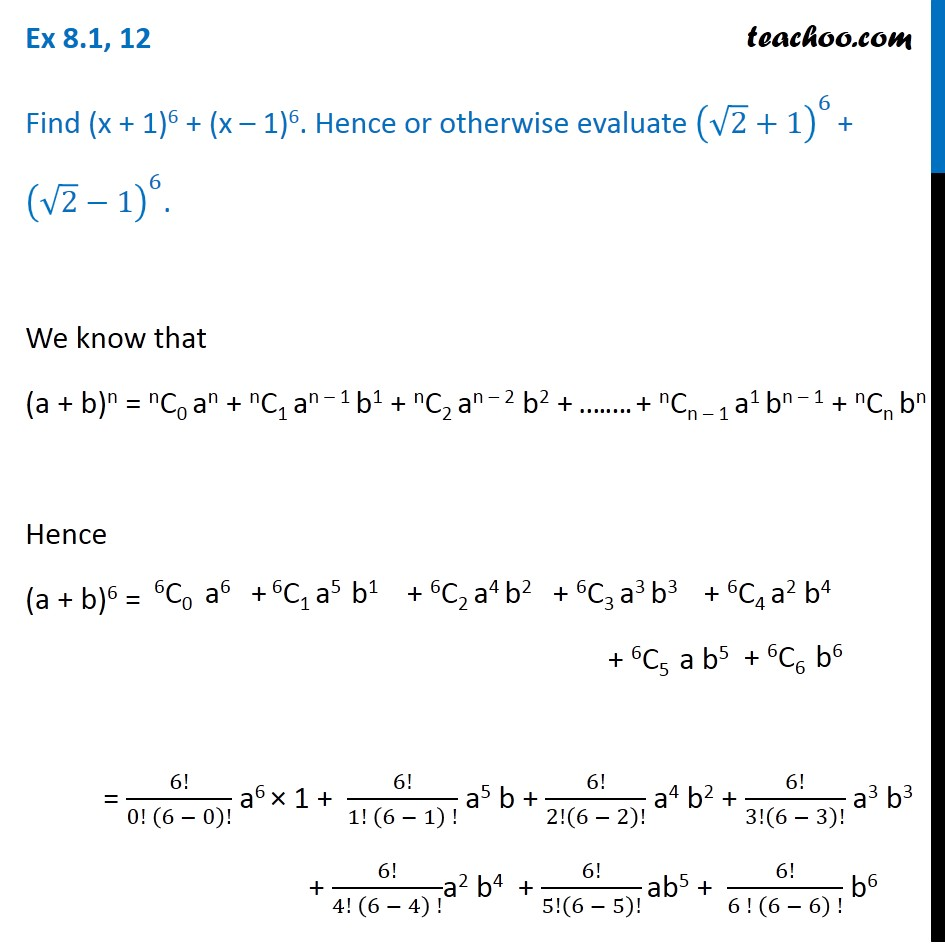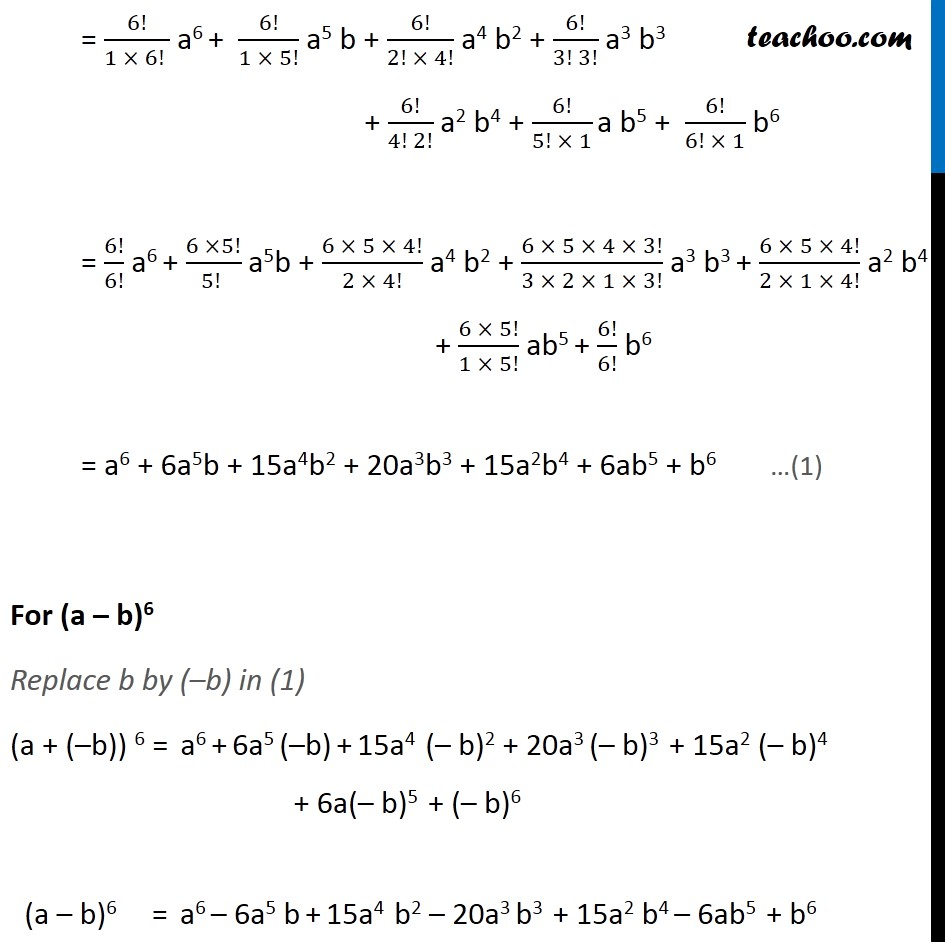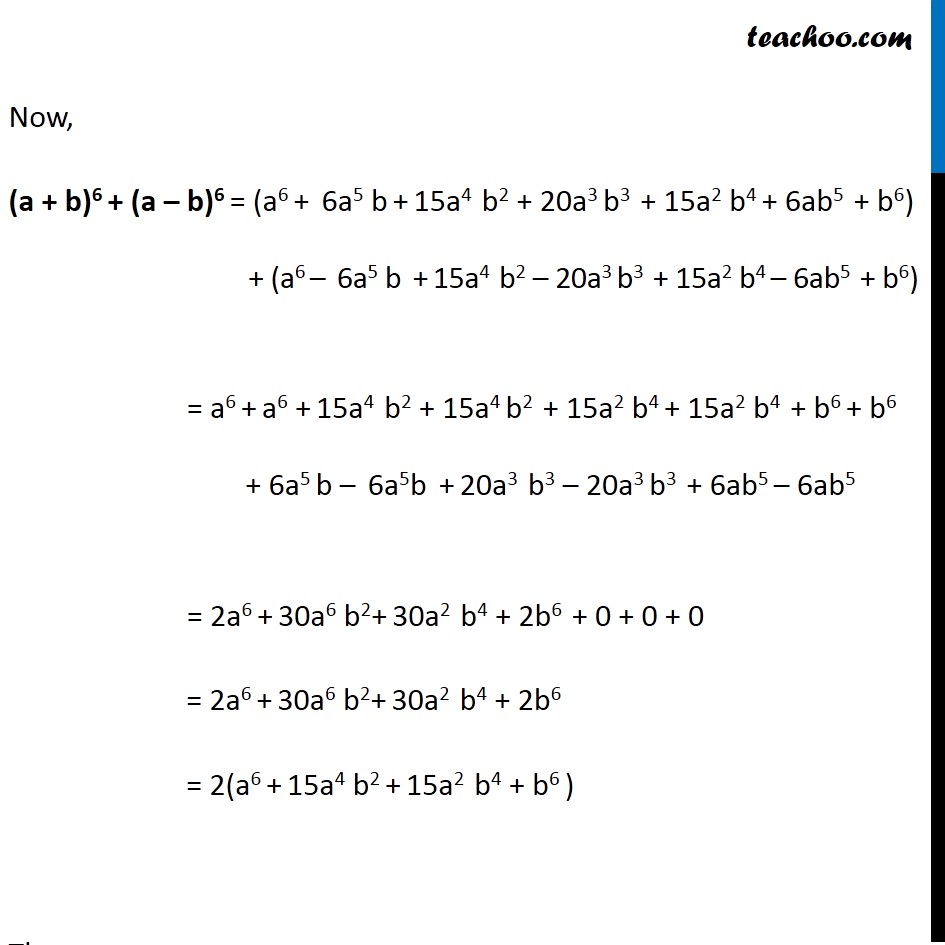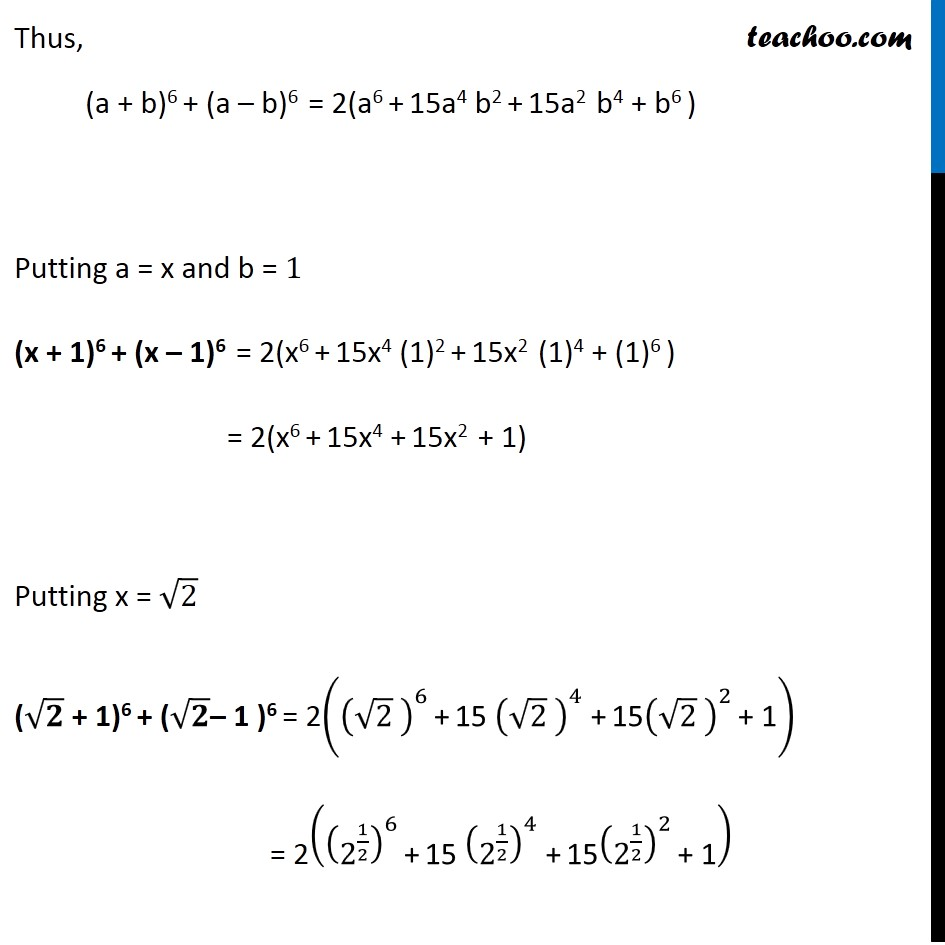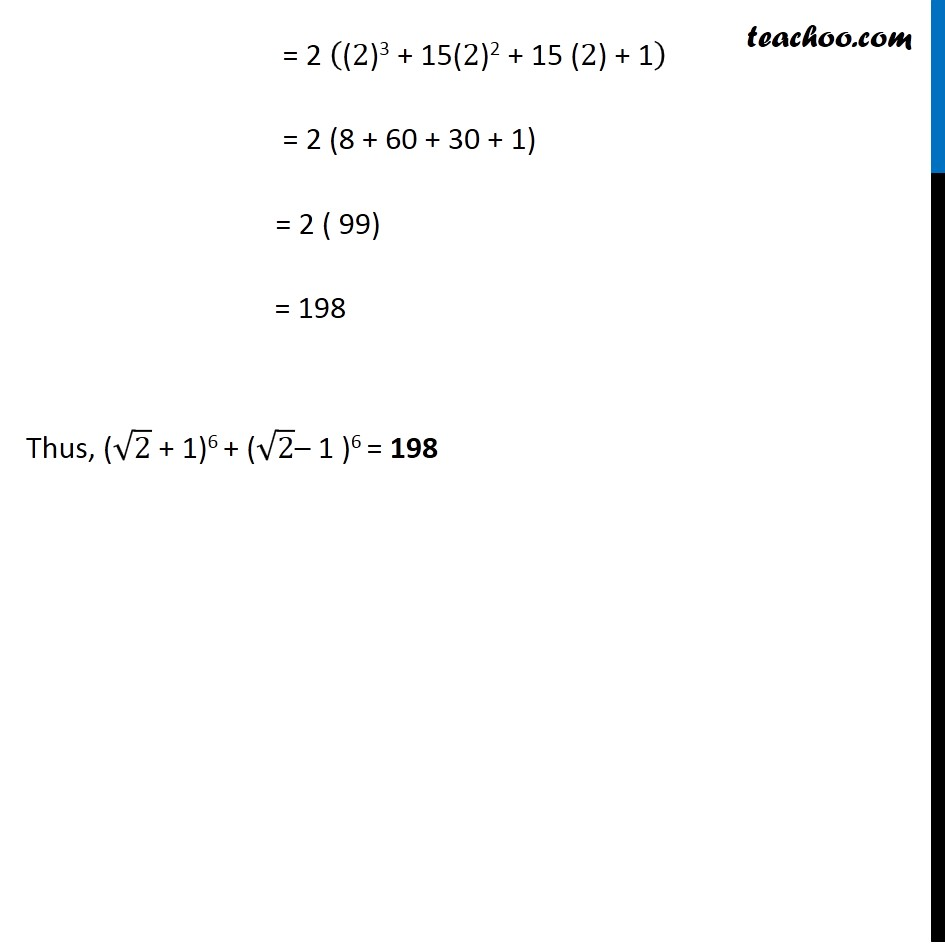### Transcript

Ex 8.1, 12 Find (x + 1)6 + (x – 1)6. Hence or otherwise evaluate (√2+1)^6 + (√2−1)^6. We know that (a + b)n = nC0 an + nC1 an – 1 b1 + nC2 an – 2 b2 + ….…. + nCn – 1 a1 bn – 1 + nCn bn Hence (a + b)6 = = 6!/(0! (6 − 0)!) a6 × 1 + 6!/(1! (6 − 1) !) a5 b + 6!/2!(6 − 2)! a4 b2 + 6!/3!(6 − 3)! a3 b3 + 6!/(4! (6 − 4) !)a2 b4 + 6!/5!(6 − 5)! ab5 + 6!/(6 ! (6 − 6) !) b6 = 6!/(1 × 6! ) a6 + 6!/(1 × 5!) a5 b + 6!/(2! × 4!) a4 b2 + 6!/(3! 3!) a3 b3 + 6!/(4! 2!) a2 b4 + 6!/(5! × 1) a b5 + 6!/(6! × 1) b6 = 6!/6! a6 + (6 ×5!)/(5! ) a5b + (6 × 5 × 4!)/(2 × 4!) a4 b2 + (6 × 5 × 4 × 3!)/(3 × 2 × 1 × 3!) a3 b3 + (6 × 5 × 4!)/(2 × 1 × 4!) a2 b4 + (6 × 5!)/(1 × 5!) ab5 + 6!/6! b6 = a6 + 6a5b + 15a4b2 + 20a3b3 + 15a2b4 + 6ab5 + b6 For (a – b)6 Replace b by (–b) in (1) (a + (–b)) 6 = a6 + 6a5 (–b) + 15a4 (– b)2 + 20a3 (– b)3 + 15a2 (– b)4 + 6a(– b)5 + (– b)6 (a – b)6 = a6 – 6a5 b + 15a4 b2 – 20a3 b3 + 15a2 b4 – 6ab5 + b6 Now, (a + b)6 + (a – b)6 = (a6 + 6a5 b + 15a4 b2 + 20a3 b3 + 15a2 b4 + 6ab5 + b6) + (a6 – 6a5 b + 15a4 b2 – 20a3 b3 + 15a2 b4 – 6ab5 + b6) = a6 + a6 + 15a4 b2 + 15a4 b2 + 15a2 b4 + 15a2 b4 + b6 + b6 + 6a5 b – 6a5b + 20a3 b3 – 20a3 b3 + 6ab5 – 6ab5 = 2a6 + 30a6 b2+ 30a2 b4 + 2b6 + 0 + 0 + 0 = 2a6 + 30a6 b2+ 30a2 b4 + 2b6 = 2(a6 + 15a4 b2 + 15a2 b4 + b6 ) Thus, (a + b)6 + (a – b)6 = 2(a6 + 15a4 b2 + 15a2 b4 + b6 ) Putting a = x and b = 1 (x + 1)6 + (x – 1)6 = 2(x6 + 15x4 (1)2 + 15x2 (1)4 + (1)6 ) = 2(x6 + 15x4 + 15x2 + 1) = 2 ("(" 2")3 + 15(" 2")2 + 15 (" 2") + 1" ) = 2 (8 + 60 + 30 + 1) = 2 ( 99) = 198 Thus, (√2 + 1)6 + (√2– 1 )6 = 198 Ex 8.1,12 Find (x + 1)6 + (x 1)6. Hence or otherwise evaluate 2 +1 6 + 2 1 6 . We know that (a + b)n = nC0 an b0 + nC1 an 1 b1 + nC2 an 2 b2 + . . + nCn 1 a1 bn 1 + nCn a0 bn Hence (a + b)6 = = 6! 0! 6 0 ! a6 1 + 6! 1 ! 6 1 ! a5 b + 6! 2! 6 2 ! a4 b2 + 6! 3 ! 6 3 ! a3 b3 + 6! 4 ! 6 4 ! a2 b4 + 6! 5 ! 6 5 ! ab5 + 6! 6 ! 6 6 ! 1 b6 = 6! 1 6! a6 + 6! 1 5! a5 b + 6! 2! 4! a4 b2 + 6! 3 ! 3! a3 b3 + 6! 4 ! 2! a2 b4 + 6! 5 ! 1 a b5 + 6! 6 ! 1 b6 = 6! 6! a6 + 6 5! 5! a5b + 6 5 4! 2 4! a4 b2 + 6 5 4 3! 3 2 1 3! a3 b3 + 6 5 4! 2 1 4! a2 b4 + 6 5! 1 5! ab5 + 6! 6! b6 = a6 + 6a5b + (3 5)a4b2 + (5 4)a3b3 + (3 5)a2b4 + 6ab5 + b6 = a6 + 6a5b + 15a4b2 + 20a3b3 + 15a2b4 + 6ab5 + b6 Thus, (a + b)6 = a6 + 6a5b + 15a4b2 + 20a3b3 + 15a2b4 + 6ab5 + b6 For (a b)6 Replace b by ( b) in (1) (a + ( b)) 6 = a6 + 6a5 ( b) + 15a4 ( b)2 + 20a3 ( b)3 + 15a2 ( b)4 + 6a( b)5 + ( b)6 (a b)6 = a6 6a5 b + 15a4 b2 20a3 b3 + 15a2 b4 6ab5 + b6 Now, (a + b)6 + (a b)6 = (a6 + 6a5 b + 15a4 b2 + 20a3 b3 + 15a2 b4 + 6ab5 + b6) + (a6 6a5 b + 15a4 b2 20a3 b3 + 15a2 b4 6ab5 + b6) = a6 + a6 + 15a4 b2 + 15a4 b2 + 15a2 b4 + 15a2 b4 + b6 + b6 + 6a5 b 6a5b + 20a3 b3 20a3 b3 + 6ab5 6ab5 = 2a6 + 30a6 b2+ 30a2 b4 + 6b6 + 0 + 0 + 0 = 2a6 + 30a6 b2+ 30a2 b4 + 6b6 = 2(a6 + 15a4 b2 + 15a2 b4 + b6 ) Thus, (a + b)6 + (a b)6 = 2(a6 + 15a4 b2 + 15a2 b4 + b6 ) Putting a = x and b = 1 (x + 1)6 + (x 1)6 = 2(x6 + 15x4 (1)2 + 15x2 (1)4 + (1)6 ) = 2(x6 + 15x4 + 15x2 + 1) Thus, (x + 1)6 + (x 1)6 = 2(x6 + 15x4 + 15x2 + 1) Putting x = 2 ( 2 + 1)6 + ( 2 1 )6 = 2 2 6 + 15 2 4 + 15 2 2 + 1 = 2 2 1 2 6 + 15 2 1 2 4 + 15 2 1 2 2 + 1 = 2 (2)3 + 15(2)2 + 15 (2) + 1 = 2 (8 + 60 + 30 + 1) = 2 ( 99) = 198 Thus, ( 2 + 1)6 + ( 2 1 )6 = 198